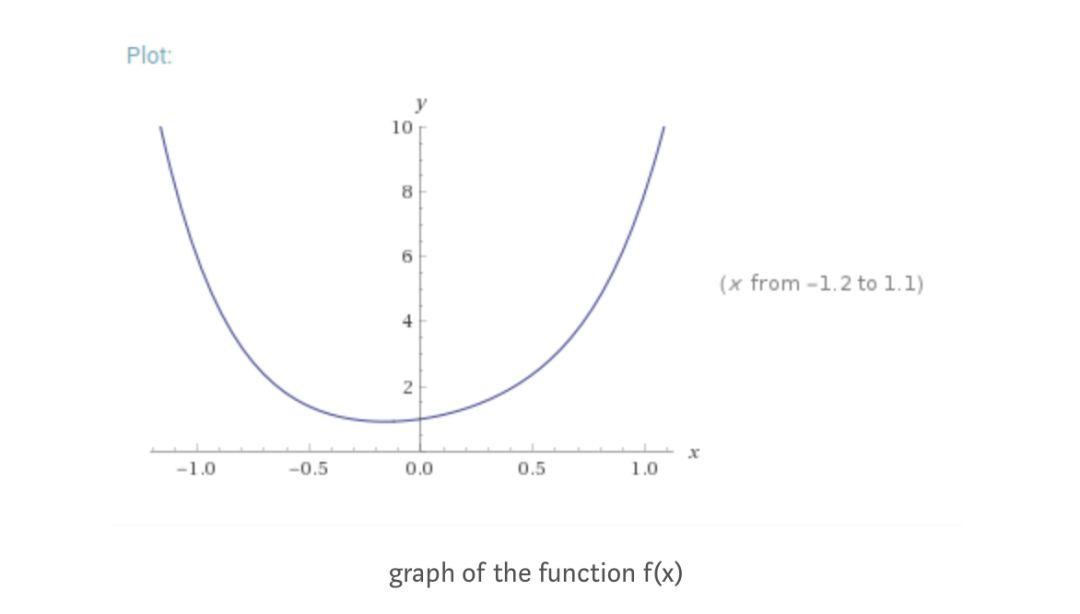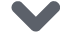# TensorFlow.js 入门指南【磐创AI导读】：本文授权转载自Tensorflow。详细介绍了Tensorflow.js的用法。想要学习更多的机器学习、深度学习的知识，欢迎大家点击上方蓝字关注我们的公众号：磐创AI

Tensorflow.js 是一个基于 deeplearn.js 构建的库，可直接在浏览器环境中创建深度学习模型。使用它可以在浏览器上创建 CNNs，RNNs 等，并使用客户端的 GPU 处理能力训练这些模型。因此，训练 NN 并不一定需要服务器级别的 GPU。本教程首先解释 TensorFlow.js 的基本构建块及其操作。然后，我们描述了如何创建一些复杂的模型。

1    <script src=”https://cdn.jsdelivr.net/npm/@tensorflow/tfjs@latest”> </script>

1    const tensor = tf.scalar(2);

1    const input = tf.tensor([2,2]);

1    const tensor_s = tf.tensor([2,2]).shape;

1    const input = tf.zeros([2,2]);

1    const a = tf.tensor([1,2,3]);

a.square().print();

TensorFlow.js 还允许链接操作。例如，要评估我们使用的张量的二次幂：

1    const x = tf.tensor([1,2,3]);

2    const x2 = x.square().square();

Tensor Disposal

1    const x = tf.tensor([1,2,3]);

2    x.dispose();

TensorFlow.js 提供了一个特殊的操作 tidy() 来自动处理中间张量：

1    function f(x)

2    {

3            return tf.tidy(()=>{

const y = x.square();

5                    const z = x.mul(y);

return z

7            });

}1    function f(x)

2    {

3        const f1 = x.pow(tf.scalar(6, ‘int32’)) //x^6

4        const f2 = x.pow(tf.scalar(4, ‘int32’)).mul(tf.scalar(2)) //2x^4

5        const f3 = x.pow(tf.scalar(2, ‘int32’)).mul(tf.scalar(3)) //3x^2

6        const f4 = tf.scalar(1) //1

8    }

1    function minimize(epochs , lr)

{

let y = tf.variable(tf.scalar(2)) //initial value

5        for(let i = 0 ; i < epochs ; i++) //start minimiziation

optim.minimize(() => f(y));

7        return y

8    }

1    xs = tf.tensor2d([[0,0],[0,1],[1,0],[1,1]])

2    ys = tf.tensor2d([,,,])

1    function createModel()

2    {

3        var model = tf.sequential()

6        model.compile({optimizer: ‘sgd’, loss: ‘binaryCrossentropy’, lr:0.1})

return model

8    }

1        await model.fit(xs, ys, {

2                batchSize: 1,

epochs: 5000

4        })

1    model.predict(xs).print()

CNN 模型

TensorFlow.js 使用计算图来自动区分。我们只需要创建图层，优化器并编译模型。让我们创建一个顺序模型：

1    model = tf.sequential();

const convlayer = tf.layers.conv2d({

inputShape: [28,28,1],

3        kernelSize: 5,

filters: 8,

5        strides: 1,

activation: ‘relu’,

7        kernelInitializer: ‘VarianceScaling’

8    });

1    const model = tf.sequential();

2

//create the first layer

5        inputShape: [28, 28, 1],

6        kernelSize: 5,

filters: 8,

8        strides: 1,

9        activation: ‘relu’,

10        kernelInitializer: ‘VarianceScaling’

11    }));

12

13    //create a max pooling layer

15        poolSize: [2, 2],

16        strides: [2, 2]

17    }));

18

19    //create the second conv layer

21        kernelSize: 5,

22        filters: 16,

23        strides: 1,

24        activation: ‘relu’,

25        kernelInitializer: ‘VarianceScaling’

26    }));

27

28    //create a max pooling layer

30        poolSize: [2, 2],

31        strides: [2, 2]

32    }));

33

34    //flatten the layers to use it for the dense layers

36

37   //dense layer with output 10 units

39    units: 10,

40        kernelInitializer: ‘VarianceScaling’,

41        activation: ‘softmax’

42    }));

1    const convlayer = tf.layers.conv2d({

inputShape: [28, 28, 1],

3        kernelSize: 5,

4        filters: 8,

5        strides: 1,

6        activation: ‘relu’,

7        kernelInitializer: ‘VarianceScaling’

8    });

9

10    const input = tf.zeros([1,28,28,1]);

11    const output = convlayer.apply(input);

const outputSize = Math.floor((inputSize-kernelSize)/stride +1);

const LEARNING_RATE = 0.0001;

1    model.compile({

2        optimizer: optimizer,

3        loss: ‘categoricalCrossentropy’,

4        metrics: [‘accuracy’],

5    });

1    const batch = tf.zeros([BATCH_SIZE,28,28,1]);

2    const labels = tf.zeros([BATCH_SIZE, NUM_CLASSES]);

3

const h = await model.fit(batch, labels,

{

6                        batchSize: BATCH_SIZE,

validationData: validationData,

8                        epochs: BATCH_EPOCHs

9                    });

10

const output = tf.oneHot(tf.tensor1d([0,1,0]), 2);

3    //the output will be [[1, 0],[0, 1],[1, 0]]

4

5

1    //h is the output of the fitting module

2    const loss = h.history.loss;

3    const accuracy = h.history.acc;

1    //retrieve the canvas

2    const canvas = document.getElementById(“myCanvas”);

const ctx = canvas.getContext(“2d”);

4

//get image data

6    imageData = ctx.getImageData(0, 0, 28, 28);

7

//convert to tensor

9    const tensor = tf.fromPixels(imageData);

const eTensor = tensor.expandDims(0);

1    model.predict(eTensor);

1    const mobilenet = await tf.loadModel(

1    //The input size is [null, 224, 224, 3]

const input_s = mobilenet.inputs.shape;

3

//The output size is [null, 1000]

5    const output_s = mobilenet.outputs.shape;

6

1    var pred = mobilenet.predict(tf.zeros([1, 224, 224, 3]));

2    pred.argMax().print();

1    //The number of layers in the model ’88’

const len = mobilenet.layers.length;

3

4    //this outputs the name of the 3rd layer ‘conv1_relu’

5    const name3 = mobilenet.layers.name;

1    const layer = mobilenet.getLayer(‘conv_pw_13_relu’);

1    mobilenet = tf.model({inputs: mobilenet.inputs, outputs: layer.output});

1    //this outputs a layer of size [null, 7, 7, 256]

const layerOutput = layer.output.shape;

1    trainableModel = tf.sequential({

2        layers: [

3            tf.layers.flatten({inputShape: [7, 7, 256]}),

4            tf.layers.dense({

units: 100,

activation: ‘relu’,

7                kernelInitializer: ‘varianceScaling’,

8                useBias: true

9            }),

10          tf.layers.dense({

11                units: 2,

12                kernelInitializer: ‘varianceScaling’,

13                useBias: false,

14                activation: ‘softmax’

15            })

16        ]

17    });

1    const activation = mobilenet.predict(input);

const predictions = trainableModel.predict(activation);

https://js.tensorflow.org/

https://github.com/tensorflow/tfjs-examples点击下方 |  | 了解更多